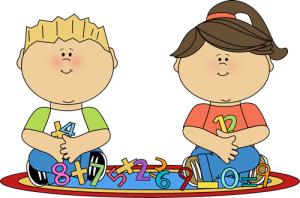## MathWe have a very interactive Math classroom! The way in which we practice Math is by practicing Math! The students are introduced to a concept and then have a chance to explore it. After exploring the concept, we begin to explain our mathematical reasoning to one another. Through these methods, the students build up a solid conceptual understanding of our topics. As well, they have opportunities to develop and refine their own thinking as opposed to practicing a formula. We learn about a variety of things such as place value, multiplication, division, fractions, geometry, problem solving, and much more!